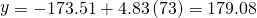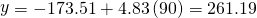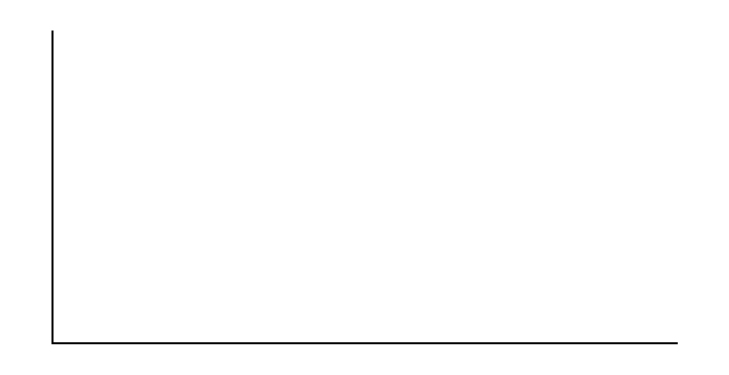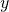Linear Regression and Correlation

# Prediction

Recall the third exam/final exam example.

We examined the scatterplot and showed that the correlation coefficient is significant. We found the equation of the best-fit line for the final exam grade as a function of the grade on the third-exam. We can now use the least-squares regression line for prediction.

Suppose you want to estimate, or predict, the mean final exam score of statistics students who received 73 on the third exam. The exam scores (x-values) range from 65 to 75. Since 73 is between the x-values 65 and 75, substitute x = 73 into the equation. Then:We predict that statistics students who earn a grade of 73 on the third exam will earn a grade of 179.08 on the final exam, on average.

Recall the third exam/final exam example.

a. What would you predict the final exam score to be for a student who scored a 66 on the third exam?

a. 145.27

b. What would you predict the final exam score to be for a student who scored a 90 on the third exam?

b. The x values in the data are between 65 and 75. Ninety is outside of the domain of the observed x values in the data (independent variable), so you cannot reliably predict the final exam score for this student. (Even though it is possible to enter 90 into the equation for x and calculate a corresponding y value, the y value that you get will not be reliable.)

To understand really how unreliable the prediction can be outside of the observed x values observed in the data, make the substitution x = 90 into the equation.The final-exam score is predicted to be 261.19. The largest the final-exam score can be is 200.

Note

The process of predicting inside of the observed x values observed in the data is called interpolation. The process of predicting outside of the observed x values observed in the data is called extrapolation.

Try It

Data are collected on the relationship between the number of hours per week practicing a musical instrument and scores on a math test. The line of best fit is as follows:

ŷ = 72.5 + 2.8x
What would you predict the score on a math test would be for a student who practices a musical instrument for five hours a week?

### References

Data from the Centers for Disease Control and Prevention.

Data from the National Center for agency reporting flu cases and TB Prevention.

Data from the United States Census Bureau. Available online at http://www.census.gov/compendia/statab/cats/transportation/motor_vehicle_accidents_and_fatalities.html

Data from the National Center for Health Statistics.

### Chapter Review

After determining the presence of a strong correlation coefficient and calculating the line of best fit, you can use the least squares regression line to make predictions about your data.

Use the following information to answer the next two exercises. An electronics retailer used regression to find a simple model to predict sales growth in the first quarter of the new year (January through March). The model is good for 90 days, where x is the day. The model can be written as follows:

ŷ = 101.32 + 2.48x where ŷ is in thousands of dollars.

What would you predict the sales to be on day 60?

💲250,120

What would you predict the sales to be on day 90?

Use the following information to answer the next three exercises. A landscaping company is hired to mow the grass for several large properties. The total area of the properties combined is 1,345 acres. The rate at which one person can mow is as follows:

ŷ = 1350 – 1.2x where x is the number of hours and ŷ represents the number of acres left to mow.

How many acres will be left to mow after 20 hours of work?

1,326 acres

How many acres will be left to mow after 100 hours of work?

How many hours will it take to mow all of the lawns? (When is ŷ = 0?)

1,125 hours, or when x = 1,125

(Figure) contains real data for the first two decades of flu cases reporting.

 Year # flu cases diagnosed # flu deaths Pre-1981 91 29 1981 319 121 1982 1,170 453 1983 3,076 1,482 1984 6,240 3,466 1985 11,776 6,878 1986 19,032 11,987 1987 28,564 16,162 1988 35,447 20,868 1989 42,674 27,591 1990 48,634 31,335 1991 59,660 36,560 1992 78,530 41,055 1993 78,834 44,730 1994 71,874 49,095 1995 68,505 49,456 1996 59,347 38,510 1997 47,149 20,736 1998 38,393 19,005 1999 25,174 18,454 2000 25,522 17,347 2001 25,643 17,402 2002 26,464 16,371 Total 802,118 489,093

Graph “year” versus “# flu cases diagnosed” (plot the scatter plot). Do not include pre-1981 data.

Perform linear regression. What is the linear equation? Round to the nearest whole number.

Check student’s solution.

Find the correlation coefficient.

1. r = ________

Solve.

1. When x = 1985, ŷ = _____
2. When x = 1990, ŷ =_____
3. When x = 1970, ŷ =______ Why doesn’t this answer make sense?
1. When x = 1985, ŷ = 25,52
2. When x = 1990, ŷ = 34,275
3. When x = 1970, ŷ = –725 Why doesn’t this answer make sense? The range of x values was 1981 to 2002; the year 1970 is not in this range. The regression equation does not apply, because predicting for the year 1970 is extrapolation, which requires a different process. Also, a negative number does not make sense in this context, where we are predicting flu cases diagnosed.

Does the line seem to fit the data? Why or why not?

What does the correlation imply about the relationship between time (years) and the number of diagnosed flu cases reported in the U.S.?

Also, the correlation r = 0.4526. If r is compared to the value in the 95% Critical Values of the Sample Correlation Coefficient Table, because r > 0.423, r is significant, and you would think that the line could be used for prediction. But the scatter plot indicates otherwise.

<!– From 12.4 Move to 12.8 –>

Plot the two given points on the following graph. Then, connect the two points to form the regression line.Obtain the graph on your calculator or computer.

<!– From 12.5 MOVE to 12.8 –>

Write the equation: ŷ= ____________= 3,448,225 + 1750x

Hand draw a smooth curve on the graph that shows the flow of the data.

<!– From 12.6 Move to 12.8 –>

Does the line seem to fit the data? Why or why not?

There was an increase in flu cases diagnosed until 1993. From 1993 through 2002, the number of flu cases diagnosed declined each year. It is not appropriate to use a linear regression line to fit to the data.

Do you think a linear fit is best? Why or why not?

What does the correlation imply about the relationship between time (years) and the number of diagnosed flu cases reported in the U.S.?

Since there is no linear association between year and # of flu cases diagnosed, it is not appropriate to calculate a linear correlation coefficient. When there is a linear association and it is appropriate to calculate a correlation, we cannot say that one variable “causes” the other variable.

<!– From 12.7 MOVE to 12.8 –>

Graph “year” vs. “# flu cases diagnosed.” Do not include pre-1981. Label both axes with words. Scale both axes.

Enter your data into your calculator or computer. The pre-1981 data should not be included. Why is that so?

Write the linear equation, rounding to four decimal places:

We don’t know if the pre-1981 data was collected from a single year. So we don’t have an accurate x value for this figure.

Regression equation: ŷ (#Flu Cases) = –3,448,225 + 1749.777 (year)

Coefficients
Intercept –3,448,225
X Variable 1 1,749.777

Find the correlation coefficient.

1. correlation = _____

### Homework

Recently, the annual number of driver deaths per 100,000 for the selected age groups was as follows:

Age Number of Driver Deaths per 100,000
16–19 38
20–24 36
25–34 24
35–54 20
55–74 18
75+ 28
1. For each age group, pick the midpoint of the interval for the x value. (For the 75+ group, use 80.)
2. Using “ages” as the independent variable and “Number of driver deaths per 100,000” as the dependent variable, make a scatter plot of the data.
3. Calculate the least squares (best–fit) line. Put the equation in the form of: ŷ = a + bx
4. Find the correlation coefficient. Is it significant?
5. Predict the number of deaths for ages 40 and 60.
6. Based on the given data, is there a linear relationship between age of a driver and driver fatality rate?
7. What is the slope of the least squares (best-fit) line? Interpret the slope.
1. Age Number of Driver Deaths per 100,000
16–19 38
20–24 36
25–34 24
35–54 20
55–74 18
75+ 28
2. Check student’s solution.
3. ŷ = 35.5818045 – 0.19182491x
4. r = –0.57874
For four df and alpha = 0.05, the LinRegTTest gives p-value = 0.2288 so we do not reject the null hypothesis; there is not a significant linear relationship between deaths and age.
Using the table of critical values for the correlation coefficient, with four df, the critical value is 0.811. The correlation coefficient r = –0.57874 is not less than –0.811, so we do not reject the null hypothesis.
5. There is not a linear relationship between the two variables, as evidenced by a p-value greater than 0.05.

(Figure) shows the life expectancy for an individual born in the United States in certain years.

Year of Birth Life Expectancy
1930 59.7
1940 62.9
1950 70.2
1965 69.7
1973 71.4
1982 74.5
1987 75
1992 75.7
2010 78.7
1. Decide which variable should be the independent variable and which should be the dependent variable.
2. Draw a scatter plot of the ordered pairs.
3. Calculate the least squares line. Put the equation in the form of: ŷ = a + bx
4. Find the correlation coefficient. Is it significant?
5. Find the estimated life expectancy for an individual born in 1950 and for one born in 1982.
6. Why aren’t the answers to part e the same as the values in (Figure) that correspond to those years?
7. Use the two points in part e to plot the least squares line on your graph from part b.
8. Based on the data, is there a linear relationship between the year of birth and life expectancy?
9. Are there any outliers in the data?
10. Using the least squares line, find the estimated life expectancy for an individual born in 1850. Does the least squares line give an accurate estimate for that year? Explain why or why not.
11. What is the slope of the least-squares (best-fit) line? Interpret the slope.

The maximum discount value of the Entertainment® card for the “Fine Dining” section, Edition ten, for various pages is given in (Figure)

Page number Maximum value (💲)
4 16
14 19
25 15
32 17
43 19
57 15
72 16
85 15
90 17
1. Decide which variable should be the independent variable and which should be the dependent variable.
2. Draw a scatter plot of the ordered pairs.
3. Calculate the least-squares line. Put the equation in the form of: ŷ = a + bx
4. Find the correlation coefficient. Is it significant?
5. Find the estimated maximum values for the restaurants on page ten and on page 70.
6. Does it appear that the restaurants giving the maximum value are placed in the beginning of the “Fine Dining” section? How did you arrive at your answer?
7. Suppose that there were 200 pages of restaurants. What do you estimate to be the maximum value for a restaurant listed on page 200?
8. Is the least squares line valid for page 200? Why or why not?
9. What is the slope of the least-squares (best-fit) line? Interpret the slope.
1. We wonder if the better discounts appear earlier in the book so we select page as X and discount as Y.
2. Check student’s solution.
3. ŷ = 17.21757 – 0.01412x
4. r = – 0.2752
For seven df and alpha = 0.05, using LinRegTTest p-value = 0.4736 so we do not reject; there is a not a significant linear relationship between page and discount.
Using the table of critical values for the correlation coefficient, with seven df, the critical value is 0.666. The correlation coefficient xi = –0.2752 is not less than 0.666 so we do not reject.
5. There is not a significant linear correlation so it appears there is no relationship between the page and the amount of the discount.

As the page number increases by one page, the discount decreases by 💲0.01412

(Figure) gives the gold medal times for every other Summer Olympics for the women’s 100-meter freestyle (swimming).

Year Time (seconds)
1912 82.2
1924 72.4
1932 66.8
1952 66.8
1960 61.2
1968 60.0
1976 55.65
1984 55.92
1992 54.64
2000 53.8
2008 53.1
1. Decide which variable should be the independent variable and which should be the dependent variable.
2. Draw a scatter plot of the data.
3. Does it appear from inspection that there is a relationship between the variables? Why or why not?
4. Calculate the least squares line. Put the equation in the form of: ŷ = a + bx.
5. Find the correlation coefficient. Is the decrease in times significant?
6. Find the estimated gold medal time for 1932. Find the estimated time for 1984.
7. Why are the answers from part f different from the chart values?
8. Does it appear that a line is the best way to fit the data? Why or why not?
9. Use the least-squares line to estimate the gold medal time for the next Summer Olympics. Do you think that your answer is reasonable? Why or why not?
State # letters in name Year entered the Union Rank for entering the Union Area (square miles)
Alabama 7 1819 22 52,423
Hawaii 6 1959 50 10,932
Iowa 4 1846 29 56,276
Maryland 8 1788 7 12,407
Missouri 8 1821 24 69,709
New Jersey 9 1787 3 8,722
Ohio 4 1803 17 44,828
South Carolina 13 1788 8 32,008
Utah 4 1896 45 84,904
Wisconsin 9 1848 30 65,499

We are interested in whether or not the number of letters in a state name depends upon the year the state entered the Union.

1. Decide which variable should be the independent variable and which should be the dependent variable.
2. Draw a scatter plot of the data.
3. Does it appear from inspection that there is a relationship between the variables? Why or why not?
4. Calculate the least-squares line. Put the equation in the form of: ŷ = a + bx.
5. Find the correlation coefficient. What does it imply about the significance of the relationship?
6. Find the estimated number of letters (to the nearest integer) a state would have if it entered the Union in 1900. Find the estimated number of letters a state would have if it entered the Union in 1940.
7. Does it appear that a line is the best way to fit the data? Why or why not?
8. Use the least-squares line to estimate the number of letters a new state that enters the Union this year would have. Can the least squares line be used to predict it? Why or why not?
1. Year is the independent or x variable; the number of letters is the dependent or y variable.
2. Check student’s solution.
3. no
4. ŷ = 47.03 – 0.0216x
5. –0.4280 The r-value indicates that there is not a significant correlation between the year the state entered the union and the number of letters in the name.
6. No, the relationship does not appear to be linear; the correlation is not significant.